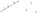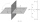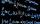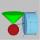# Curve and line

The equation of a curve C is y=2x² -8x+9 and the equation of a line L is x+ y=3

(1) Find the x co-ordinates of the points of intersection of L and C.
(2) Show that one of these points is also the stationary point of C?

Result

a1 =  2
a2 =  1.5
b =  2

#### Solution:Checkout calculation with our calculator of quadratic equations.Leave us a comment of example and its solution (i.e. if it is still somewhat unclear...):

Showing 0 comments:Be the first to comment!#### To solve this example are needed these knowledge from mathematics:

For Basic calculations in analytic geometry is helpful line slope calculator. From coordinates of two points in the plane it calculate slope, normal and parametric line equation(s), slope, directional angle, direction vector, the length of segment, intersections the coordinate axes etc. Looking for help with calculating roots of a quadratic equation?

## Next similar examples:

1. Parametric equationFind the parametric equation of a line with y-intercept (0,-4) and a slope of -2.
2. Points collinearShow that the point A(-1,3), B(3,2), C(11,0) are col-linear.
3. Symmetry by planeDetermine the coordinates of a image of point A (3, -4, -6) at a symmetry that is determined by the plane x-y-4z-13 = 0
4. LineIt is true that the lines that do not intersect are parallel?
5. LineLine p passing through A[-10, 6] and has direction vector v=(3, 2). Is point B[7, 30] on the line p?
6. Quadratic equationFind the roots of the quadratic equation: 3x2-4x + (-4) = 0.
7. Quadratic function 2Which of the points belong function f:y= 2x2- 3x + 1 : A(-2, 15) B (3,10) C (1,4)
8. RootsDetermine the quadratic equation absolute coefficient q, that the equation has a real double root and the root x calculate: ?
9. DiscriminantDetermine the discriminant of the equation: ?
10. EquationEquation ? has one root x1 = 8. Determine the coefficient b and the second root x2.
11. CombinationsHow many elements can form six times more combinations fourth class than combination of the second class?
12. Solve 3Solve quadratic equation: (6n+1) (4n-1) = 3n2
13. Variations 4/2Determine the number of items when the count of variations of fourth class without repeating is 600 times larger than the count of variations of second class without repetition.
14. CombinationsFrom how many elements we can create 990 combinations 2nd class without repeating?
15. Minimum of sumFind a positive number that the sum of the number and its inverted value was minimal.
16. ConeInto rotating cone with dimensions r = 8 cm and h = 8 cm incribe cylinder with maximum volume so that the cylinder axis is perpendicular to the axis of the cone. Determine the dimensions of the cylinder.
17. Theorem proveWe want to prove the sentense: If the natural number n is divisible by six, then n is divisible by three. From what assumption we started?